Courses

# Test: Circles -1

## 10 Questions MCQ Test Mathematics (Maths) Class 11 | Test: Circles -1

Description
This mock test of Test: Circles -1 for JEE helps you for every JEE entrance exam. This contains 10 Multiple Choice Questions for JEE Test: Circles -1 (mcq) to study with solutions a complete question bank. The solved questions answers in this Test: Circles -1 quiz give you a good mix of easy questions and tough questions. JEE students definitely take this Test: Circles -1 exercise for a better result in the exam. You can find other Test: Circles -1 extra questions, long questions & short questions for JEE on EduRev as well by searching above.
QUESTION: 1

### The equation of parabola whose focus is (– 3, 0) and directrix x + 5 = 0 is:

Solution: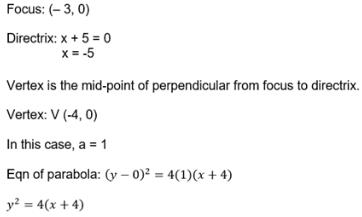QUESTION: 2

### The equation of the circle passing through (0, 0) and making intercepts 2 and 4 on the coordinate axes is:

Solution: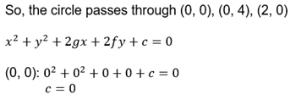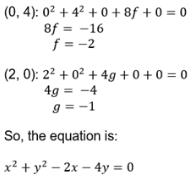QUESTION: 3

### A circle is the set of …… in a plane that are equidistant from a fixed point in the plane.

Solution:

A circle is the set of points a fixed distance from a center point,

QUESTION: 4

The centre and radius of the circle x2 + y2 + 4x – 6y = 5 is:

Solution:

x2+y2+4x-6y=5
Circle Equation
(x-a)2+(y-b)2=r2 is the circle equation with a radius r, centered at (a,b)
Rewrite x2+y24x-6y=5 in the form of circle standard circle equation
(x-(-2))2+(y-3)2=(3√2)2
Therefore the circle properties are:

(a,b) = (-2,3), r = 3√2

QUESTION: 5

The equation of a circle with centre as the origin is

Solution: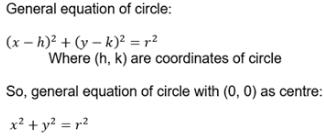QUESTION: 6

The equation of circle whose centre is (2, 1) and which passes through the point (3, – 5) is:

Solution:
QUESTION: 7

The equation of circle of radius 5 units touches the coordinates axes in the second quadrant is:

Solution: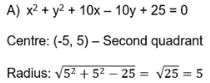QUESTION: 8

The equation of the circle passing through (0, 0) and making intercepts a and b on the coordinate axes is:

Solution:

Let the equation of the required circle be (x – h)2 + (y – k)2 = r2.
Since the centre of the circle passes through (0, 0),
(0 – h)2 + (0 – k)2 = r2
⇒ h2 + k2 = r2
The equation of the circle now becomes (x – h)2 + (y – k)2 = h2 + k2.
It is given that the circle makes intercepts a and b on the coordinate axes. This means that the circle passes through points (a, 0) and (0, b). Therefore,
(a – h)2 + (0 – k)2 = h2 + k2 …………… (1)
(0 – h)2 + (b – k)2 = h2 + k2 ………… (2)
From equation (1), we obtain a2 – 2ah + h2 + k2 = h2 + k2
⇒ a2 – 2ah = 0
⇒ a(a – 2h) = 0
⇒ a = 0 or (a – 2h) = 0
However, a ≠ 0; hence, (a – 2h) = 0 ⇒ h =a/2.
From equation (2), we obtain h2 + b2 – 2bk + k2 = h2 + k2
⇒ b2 – 2bk = 0
⇒ b(b – 2k) = 0
⇒ b = 0 or(b – 2k) = 0
However, b ≠ 0; hence, (b – 2k) = 0 ⇒ k =b/2. Thus, the equation of the required circle is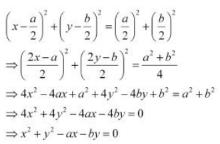QUESTION: 9

The equation of a circle which is concentric to the given circle x2 + y2 - 4x - 6y - 3 = 0 and which touches the X-axis is:

Solution:

x² + y² -4x -6y -3 = 0

(x² -4x +4 -4) + (y² -6y +9-9) = 3

(x²-4x +4) +(y²-6y+9) = 3+4+9

(x-2) ² +(y-3) ²=16

This is circle with center(2,3) and radius 4

Since required circle is concentric to this circle

So its center will be (2,3)

Let circle be

(x-2) ²+(y-3) ²=r²…………(1)

Now we need radius of this circle.

As we are also given that circle touches y axis

So y axis is tangent to the circle.

Point of contact will be (0,3)

Let’s substitute (0,3) in circle (1)

(0-2) ² + (3-3) ²=r²

So radius is 2 as r²=4

So eqn of the circle becomes

(x-2) ²+(y-3) ²=4

x² +y² -4x-6x+4+9=4

x² +y² -4x-6x+9=0

QUESTION: 10

Point (-2, – 5) lies on the circle x2 + y2 = 25.

Solution: